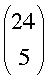SEARCH HOMEMath Central Quandaries & QueriesQuestion from samhita, a student: Find the number of ways of arranging 26 letters in the English alphabets in a row such that there are exactly 5 letters between x and y.We have two responses for you

Hello Samhita.

Start by removing the letters x and y from the alphabet. This leaves 24 letters. How many ways can you arrange 24 letters? The answer to that is 24! of course.

Now given any permutation taken from the above orderings of 24 letters, we will insert the x and the y. For now, let's say the x comes before the y. How many places can the x go, if the y is separated from it by 5 other letters? Certainly, x could be in position 1 and y in position 7, or x in pos. 2 and y in pos. 8. This works up to x in position 20 and y in position 26. Thus there are 20 possible ways of inserting the x and y such that the x precedes the y. But since the y could precede the x, there are an additional 20 ways of inserting the two letters into the 24.

Thus, there are 40 ways of inserting the x and y into each of 24! permutations of the other letters.

Hope this helps,
Stephen La Rocque.

Samhita,

I would start by choosing 5 letters to place between x and y. I can do this inways. I can then rearrange the order of the 5 letters in 5! ways and place them between x and y with either x at the start or y at the start. Thus I can construct this string of 7 letters in× 5! × 2 ways.

Finally you can arrange the 19 remaining letters and the string of 7 letters including x and y in 20! ways.

PennyMath Central is supported by the University of Regina and The Pacific Institute for the Mathematical Sciences.